# Sharpe Ratio

## Distribution of Maximal Sharpe, Corrected Bonferroni

In a previous blog post we used the 'Polyhedral Inference' trick of Lee et al. to perform conditional inference on the asset with maximum Sharpe ratio. This is now a short paper on arxiv. I was somewhat disappointed to find, as noted in the paper, that polyhedral inference has lower power than a simple Bonferroni correction against alternatives where many assets have the same Signal-Noise ratio. (Though apparently it has higher power when one asset alone higher SNR.) The interpretation is that when there is no spread in the SNR, Bonferroni correction should have the same power as a single asset test, while conditional inference is sensitive to the conditioning information that you are testing a single asset which has Sharpe ratio perhaps near that of other assets. In the opposite case, Bonferroni suffers from having to 'pay' for a lot of irrelevant (for having low Sharpe) assets, while conditional inference does fine.

I also showed in the paper, as I demonstrated in a previous blog post, that the Bonferroni correction is conservative when asset returns are correlated. In a simple simulations under the null, I showed that the empirical type I rate goes to zero as common correlation $$\rho$$ goes to one. In this blog post I will describe a simple trick to correct for average positive correlation.

So let us suppose that we observe returns on $$p$$ assets over $$n$$ days, and that returns have correlation matrix $$R$$. Let $$\hat{\zeta}$$ be the vector of Sharpe ratios over this sample. In the paper I show that if returns are normal then the following approximation holds

$$\hat{\zeta}\approx\mathcal{N}\left(\zeta,\frac{1}{n}\left( R + \frac{1}{2}\operatorname{Diag}\left(\zeta\right)\left(R \odot R\right)\operatorname{Diag}\left(\zeta\right) \right)\right).$$

There is a more general form for Elliptically distributed returns. In the paper I find, via simulations, that for realistic SNRs and large sample sizes, the more general form does not add much accuracy. In fact, for the small SNRs one is likely to see in practice the simple approximation

$$\hat{\zeta}\approx\mathcal{N}\left(\zeta,\frac{1}{n}R\right)$$

will suffice.

Now note that, under the null hypothesis that $$\zeta = \zeta_0$$, one has

$$z = \sqrt{n} \left(R^{1/2}\right)^{-1} \left(\hat{\zeta} - \zeta_0\right) \approx\mathcal{N}\left(0,I\right),$$

where $$R^{1/2}$$ is a matrix square root of $$R$$. Testing the null hypothesis should proceed by computing (or estimating) the vector $$z$$, then comparing to normality, either by a Chi-square statistic, or performing Bonferroni-corrected normal inference on the largest element.

In the paper I used a simple rank-one model for correlation for simulations using

$$R = \left(1-\rho\right) I + \rho 1 1^{\top}.$$

This effectively models the influence of a common single 'latent' factor. Certainly this is more flexible for modeling real returns than assuming identity correlation, but is not terribly realistic.

Under this model of $$R$$ it is simple enough to compute the inverse-square-root of $$R$$. Namely

$$\left(R^{1/2}\right)^{-1} = \left(1-\rho\right)^{-1/2} I + \frac{1}{p}\left(\frac{1}{\sqrt{1-\rho+p\rho}} - \frac{1}{\sqrt{1-\rho}}\right)1 1^{\top}.$$

Let's just confirm with code:

p <- 4
rho <- 0.3
R <- (1-rho) * diag(p) + rho
ihR <- (1/sqrt(1-rho)) * diag(p) + (1/p) * ((1/sqrt(1-rho+p*rho)) - (1/sqrt(1-rho)))
hR <- solve(ihR)
R - hR %*% hR

##             [,1]        [,2]        [,3]        [,4]
## [1,] 4.44089e-16 1.66533e-16 1.11022e-16 1.66533e-16
## [2,] 1.66533e-16 0.00000e+00 5.55112e-17 5.55112e-17
## [3,] 1.66533e-16 1.66533e-16 0.00000e+00 1.11022e-16
## [4,] 1.11022e-16 1.11022e-16 1.11022e-16 2.22045e-16


So to test the null hypothesis, one computes

$$z = \sqrt{n} \left( \left(1-\rho\right)^{-1/2} I + \frac{1}{p}\left(\frac{1}{\sqrt{1-\rho+p\rho}} - \frac{1}{\sqrt{1-\rho}}\right)1 1^{\top} \right) \left(\hat{\zeta} - \zeta_0\right)$$

to test against normality. But note that our linear transformation is monotonic (indeed affine): if $$v_i \ge v_j$$ and $$w = \left(R^{1/2}\right)^{-1} v$$, then $$w_i \ge w_j$$. This means that the maximum element of $$z$$ has the same index as the maximum element of $$\hat{\zeta} - \zeta_0$$. To perform Bonferroni correction we need only transform the largest element of $$\hat{\zeta} - \zeta_0$$, by scaling it up, and shifting to accomodate the average. So if the largest element of $$\hat{\zeta} - \zeta_0$$ is $$y$$, and the average value is $$a = \frac{1}{p}1^{\top} \left(\hat{\zeta} - \zeta_0\right)$$, then the largest value of $$z$$ is

$$\frac{\sqrt{n} y}{\sqrt{1-\rho}} + a \sqrt{n} \left(\frac{1}{\sqrt{1-\rho+p\rho}} - \frac{1}{\sqrt{1-\rho}}\right)$$

Reject the null hypothesis if this is larger than $$\Phi\left(1 - \alpha/p\right)$$.

## Simulations

Here we perform simple simulations of Bonferroni and corrected Bonferroni. We will assume that returns are Gaussian, that the correlation follows our simple rank one form, that the correlation is known in order to perform the corrected test. We simulate two years of daily data on 100 assets. For each choice of $$\rho$$ we perform 10000 simulations under the null of zero SNR, computing the simple and 'improved' Bonferroni corrected hypothesis tests. We tabulate the empirical type I rate and plot against $$\rho$$.

suppressMessages({
library(dplyr)
library(tidyr)
library(doFuture)
})
# set up the functions
rawsim <- function(nday,nlatf,nsim=100,rho=0) {
R <- pmin(diag(nlatf) + rho,1)
mu <- rep(0,nlatf)

apart <- sqrt(nday)/sqrt(1-rho)
bpart <- sqrt(nday) * ((1/sqrt(1-rho+nlatf*rho)) - (1/sqrt(1-rho)))

mhtpvals <- replicate(nsim,{
X <- mvtnorm::rmvnorm(nday,mean=mu,sigma=R)
x <- colMeans(X) / apply(X,2,sd)
bonf_pval <- nlatf * SharpeR::psr(max(x),df=nday-1,zeta=0,ope=1,lower.tail=FALSE)
# do the correction
corr_stat <- apart * max(x) + bpart * mean(x)
corr_pval <- nlatf * pnorm(corr_stat,lower.tail=FALSE)

c(bonf_pval,corr_pval)
})
data_frame(bonf_pvals=as.numeric(mhtpvals[1,]),
corr_pvals=as.numeric(mhtpvals[2,]))
}
many_rawsim <- function(nday,nlatf,rho,nsim=1000L,nnodes=7) {
if ((nsim > 10*nnodes) && require(doFuture)) {
registerDoFuture()
plan(multiprocess)
nper <- as.numeric(table(1:nsim %% nnodes))
retv <- foreach(iii=1:nnodes,.export = c('nday','nlatf','rho','nper','rawsim')) %dopar% {
rawsim(nday=nday,nlatf=nlatf,rho=rho,nsim=nper[iii])
} %>%
bind_rows()
} else {
retv <- rawsim(nday=nday,nlatf=nlatf,rho=rho,nsim=nsim)
}
retv
}
mhtsim <- function(alpha=0.05,...) {
many_rawsim(...) %>%
tidyr::gather(key=method,value=pvalues) %>%
group_by(method) %>%
summarize(rej_rate=mean(pvalues < alpha)) %>%
ungroup() %>%
arrange(method)
}

# perform simulations
nsim <- 10000
nday <- 2*252
nlatf <- 100

params <- data_frame(rho=seq(0.01,0.99,length.out=7))

set.seed(123)
resu <- params %>%
group_by(rho) %>%
summarize(resu=list(mhtsim(nday=nday,nlatf=nlatf,rho=rho,nsim=nsim))) %>%
ungroup() %>%
unnest()

suppressMessages({
library(dplyr)
library(ggplot2)
})
# plot empirical rates
ph <- resu %>%
mutate(method=gsub('bonf_pvals','Plain Bonferroni',method)) %>%
mutate(method=gsub('corr_pvals','Corrected Bonferroni',method)) %>%
ggplot(aes(rho,rej_rate,color=method)) +
geom_line() + geom_point() +
geom_hline(yintercept=0.05,linetype=2,alpha=0.5) +
scale_y_sqrt() +
labs(title='Empirical type I rate at the 0.05 level',
x=expression(rho),y='type I rate',
color='test')
print(ph)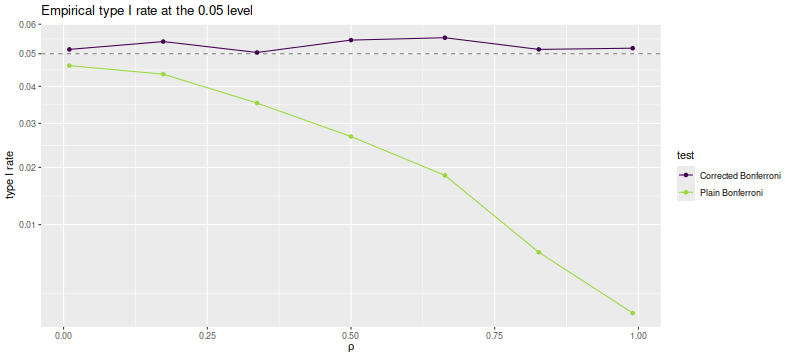As desired, we maintain nominal coverage using the correction for $$\rho$$, while the naive Bonferroni is too conservative for large $$\rho$$. This is not yet a practical test, but could be used for rough estimation by plugging in the average sample correlation (or just SWAG'ing one). To my tastes a more interesting question is whether one can generalize this process to a rank $$k$$ approximation of $$R$$ while keeping the monotonicity property. (I have my doubts this is possible)

## Distribution of Maximal Sharpe, Truncated Normal

In a previous blog post we looked at a symmetric confidence intervals on the Signal-Noise ratio. That study was motivated by the "opportunistic strategy", wherein one observes the historical returns of an asset to determine whether to hold it long or short. Then, conditional on the sign of the trade, we were able to construct proper confidence intervals on the Signal-Noise ratio of the opportunistic strategy's returns.

I had hoped that one could generalize from the single asset opportunistic strategy to the case of $$p$$ assets, where one constructs the Markowitz portfolio based on observed returns. I have not had much luck finding that generalization. However, we can generalize the opportunistic strategy in a different way to what I call the "Winner Take All" strategy. Here one observes the historical returns of $$p$$ different assets, then chooses the one with the highest observed Sharpe ratio to hold long. (Let us hold off on an Opportunistic Winner Take All.)

Observe, however, this is just the problem of inferring the Signal Noise ratio (SNR) of the asset with the maximal Sharpe. We previously approached that problem using a Markowitz approximation, finding it somewhat lacking. That Markowitz approximation was an attempt to correct some deficiencies with what is apparently the state of the art in the field, Marcos Lopez de Prado's (now AQR's?) "Most Important Plot in All of Finance", which is a thin layer of Multiple Hypothesis Testing correction over the usual distribution of the Sharpe ratio. In a previous blog post, we found that Lopez de Prado's method would have lower than nominal type I rates as it ignored correlation of assets.

Moreover, a simple MHT correction will not, I think, deal very well with the case where there are great differences in the Signal Noise ratios of the assets. The 'stinker' assets with low SNR will simply spoil our inference, unlikely to have much influence on which asset shows the highest Sharpe ratio, and only causing us to increase our significance threshold.

With my superior googling skills I recently discovered a 2013 paper by Lee et al, titled Exact Post-Selection Inference, with Application to the Lasso. While aimed at the Lasso, this paper includes a procedure that essentially solves our problem, giving hypothesis tests or confidence intervals with nominal coverage on the asset with maximal Sharpe among a set of possibly correlated assets.

The Lee et al paper assumes one observes $$p$$-vector

$$y \sim \mathcal{N}\left(\mu,\Sigma\right).$$

Then conditional on $$y$$ falling in some polyhedron, $$Ay \le b$$, we wish to perform inference on $$\nu^{\top}y$$. In our case the polyhedron will be the union of all polyhedra with the same maximal element of $$y$$ as we observed. That is, assume that we have reordered the elements of $$y$$ such that $$y_1$$ is the largest element. Then $$A$$ will be a column of negative ones, cbinded to the $$p-1$$ identity matrix, and $$b$$ will be a $$p-1$$ vector of zeros. The test is defined on $$\nu=e_1$$ the vector with a single one in the first element and zero otherwise.

Their method works by decomposing the condition $$Ay \le b$$ into a condition on $$\nu^{\top}y$$ and a condition on some $$z$$ which is normal but independent of $$\nu^{\top}y$$. You can think of this as kind of inverting the transform by $$A$$. After this transform, the value of $$\nu^{\top}y$$ is restricted to a line segment, so we need only perform inference on a truncated normal.

The code to implement this is fairly straightforward, and given below. The procedure to compute the quantile function, which we will need to compute confidence intervals, is a bit trickier, due to numerical issues. We give a hacky version below.

# Lee et. al eqn (5.8)
F_fnc <- function(x,a,b,mu=0,sigmasq=1) {
sigma <- sqrt(sigmasq)
phis <- pnorm((c(x,a,b)-mu)/sigma)
(phis - phis) / (phis - phis)
}
# Lee eqns (5.4), (5.5), (5.6)
Vfuncs <- function(z,A,b,ccc) {
Az <- A %*% z
Ac <- A %*% ccc
bres <- b - Az
brat <- bres
brat[Ac!=0] <- brat[Ac!=0] / Ac[Ac!=0]
Vminus <- max(brat[Ac < 0])
Vplus  <- min(brat[Ac > 0])
Vzero  <- min(bres[Ac == 0])
list(Vminus=Vminus,Vplus=Vplus,Vzero=Vzero)
}
# Lee et. al eqn (5.9)
ptn <- function(y,A,b,nu,mu,Sigma,numu=as.numeric(t(nu) %*% mu)) {
Signu <- Sigma %*% nu
nuSnu <- as.numeric(t(nu) %*% Signu)
ccc <- Signu / nuSnu  # eqn (5.3)
nuy <- as.numeric(t(nu) %*% y)
zzz <- y - ccc * nuy  # eqn (5.2)
Vfs <- Vfuncs(zzz,A,b,ccc)
F_fnc(x=nuy,a=Vfs$Vminus,b=Vfs$Vplus,mu=numu,sigmasq=nuSnu)
}
# invert the ptn function to find nu'mu at a given pval.
citn <- function(p,y,A,b,nu,Sigma) {
Signu <- Sigma %*% nu
nuSnu <- as.numeric(t(nu) %*% Signu)
ccc <- Signu / nuSnu  # eqn (5.3)
nuy <- as.numeric(t(nu) %*% y)
zzz <- y - ccc * nuy  # eqn (5.2)
Vfs <- Vfuncs(zzz,A,b,ccc)

# you want this, but there are numerical issues:
#f <- function(numu) { F_fnc(x=nuy,a=Vfs$Vminus,b=Vfs$Vplus,mu=numu,sigmasq=nuSnu) - p }
sigma <- sqrt(nuSnu)
f <- function(numu) {
phis <- pnorm((c(nuy,Vfs$Vminus,Vfs$Vplus)-numu)/sigma)
#(phis - phis) - p * (phis - phis)
phis - (1-p) * phis - p * phis
}
# this fails sometimes, so find a better interval
intvl <- c(-1,1)  # a hack.
# this is very unfortunate
trypnts <- seq(from=min(y),to=max(y),length.out=31)
ys <- sapply(trypnts,f)
dsy <- diff(sign(ys))
if (any(dsy < 0)) {
widx <- which(dsy < 0)
intvl <- trypnts[widx + c(0,1)]
} else {
maby <- 2 * (0.1 + max(abs(y)))
trypnts <- seq(from=-maby,to=maby,length.out=31)
ys <- sapply(trypnts,f)
dsy <- diff(sign(ys))
if (any(dsy < 0)) {
widx <- which(dsy < 0)
intvl <- trypnts[widx + c(0,1)]
}
}
uniroot(f=f,interval=intvl,extendInt='yes')$root }  ## Testing on normal data Here we test the code above on the problem considered in Theorem 5.2 of Lee et al. That is, we draw $$y \sim \mathcal{N}\left(\mu,\Sigma\right)$$, then observe the value of $$F$$ given in Theorem 5.2 when we plug in the actual population values of $$\mu$$ and $$\Sigma$$. This is several steps removed from our problem of inference on the SNR, but it is best to pause and make sure the implementation is correct first. We perform 5000 simulations, letting $$p=20$$, then creating a random $$\mu$$ and $$\Sigma$$, drawing a single $$y$$, observing which element is the maximum, creating $$A, b, \nu$$, then computing the $$F$$ function, resulting in a $$p$$-value which should be uniform. We Q-Q plot those empirical $$p$$-values against a uniform law, finding them on the $$y=x$$ line. gram <- function(x) { t(x) %*% x } rWish <- function(n,p=n,Sigma=diag(p)) { require(mvtnorm) gram(rmvnorm(p,sigma=Sigma)) } nsim <- 5000 p <- 20 A1 <- cbind(-1,diag(p-1)) set.seed(1234) pvals <- replicate(nsim,{ mu <- rnorm(p) Sigma <- rWish(n=2*p+5,p=p) y <- t(rmvnorm(1,mean=mu,sigma=Sigma) ) # collect the maximum, so reorder the A above yord <- order(y,decreasing=TRUE) revo <- seq_len(p) revo[yord] <- revo A <- A1[,revo] nu <- rep(0,p) nu[yord] <- 1 b <- rep(0,p-1) foo <- ptn(y=y,A=A,b=b,nu=nu,mu=mu,Sigma=Sigma) }) # plot them library(dplyr) library(ggplot2) ph <- data_frame(pvals=pvals) %>% ggplot(aes(sample=pvals)) + geom_qq(distribution=stats::qunif) + geom_qq_line(distribution=stats::qunif) print(ph)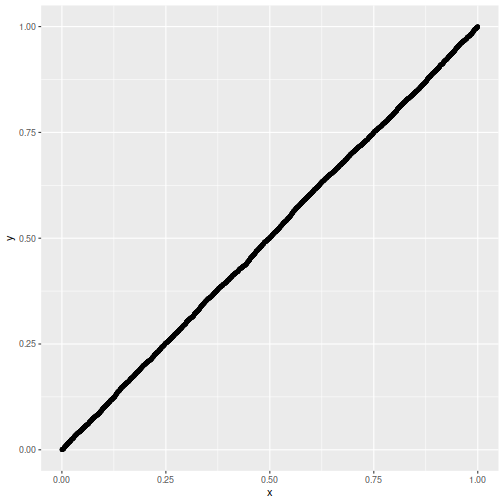Now we attempt to use the confidence interval code. We construct a one-sided 95% confidence interval, and check how often it is violated by the $$\mu$$ of the element which shows the highest $$y$$. We will find that the empirical rate of violations of our confidence interval is indeed around 5%: nsim <- 5000 p <- 20 A1 <- cbind(-1,diag(p-1)) set.seed(1234) tgtval <- 0.95 viols <- replicate(nsim,{ mu <- rnorm(p) Sigma <- rWish(n=2*p+5,p=p) y <- t(rmvnorm(1,mean=mu,sigma=Sigma) ) # collect the maximum, so reorder the A above yord <- order(y,decreasing=TRUE) revo <- seq_len(p) revo[yord] <- revo A <- A1[,revo] nu <- rep(0,p) nu[yord] <- 1 b <- rep(0,p-1) # mu is unknown to this guy foo <- citn(p=tgtval,y=y,A=A,b=b,nu=nu,Sigma=Sigma) violated <- mu[yord] < foo }) print(sprintf('%.2f%%',100*mean(viols)))  ##  "5.04%"  ## Testing on the Sharpe ratio To use this machinery to perform inference on the SNR, we can either port the results to the multivariate $$t$$-distribution, which seems unlikely because uncorrelated marginals of a multivariate $$t$$ are not independent. Instead we lean on the normal approximation to the vector of Sharpe ratios. If the $$p$$-vector $$x$$ is normal with correlation matrix $$R$$, then $$\hat{\zeta}\approx\mathcal{N}\left(\zeta,\frac{1}{n}\left( R + \frac{1}{2}\operatorname{Diag}\left(\zeta\right)\left(R \odot R\right)\operatorname{Diag}\left(\zeta\right) \right)\right),$$ where $$\hat{\zeta}$$ is the $$p$$-vector of Sharpe ratios computed by observing $$n$$ independent draws of $$x$$, and $$\zeta$$ is the $$p$$-vector of Signal Noise ratios. Note how this generalizes the 'Lo' form of the standard error of a scalar Sharpe ratio, viz $$\sqrt{(1 + \zeta^2/2)/n}$$. Here we will check the uniformity of $$p$$ values resulting from using this normal approximation. This is closer to the actual inference we want to do, except we will cheat by using the actual $$R$$ and $$\zeta$$ to construct what is essentially the $$\Sigma$$ to Lee's formulation. We will set $$p=20$$ and draw 3 years of daily data. Again we plot the putative $$p$$-values against uniformity and find a good match. # let's test it! nsim <- 5000 p <- 20 ndays <- 3 * 252 A1 <- cbind(-1,diag(p-1)) set.seed(4321) pvals <- replicate(nsim,{ # population values here mu <- rnorm(p) Sigma <- rWish(n=2*p+5,p=p) RRR <- cov2cor(Sigma) zeta <- mu /sqrt(diag(Sigma)) Xrets <- rmvnorm(ndays,mean=mu,sigma=Sigma) srs <- colMeans(Xrets) / apply(Xrets,2,FUN=sd) y <- srs mymu <- zeta mySigma <- (1/ndays) * (RRR + (1/2) * diag(zeta) %*% (RRR * RRR) %*% diag(zeta)) # collect the maximum, so reorder the A above yord <- order(y,decreasing=TRUE) revo <- seq_len(p) revo[yord] <- revo A <- A1[,revo] nu <- rep(0,p) nu[yord] <- 1 b <- rep(0,p-1) foo <- ptn(y=y,A=A,b=b,nu=nu,mu=mymu,Sigma=mySigma) }) # plot them library(dplyr) library(ggplot2) ph <- data_frame(pvals=pvals) %>% ggplot(aes(sample=pvals)) + geom_qq(distribution=stats::qunif) + geom_qq_line(distribution=stats::qunif) print(ph)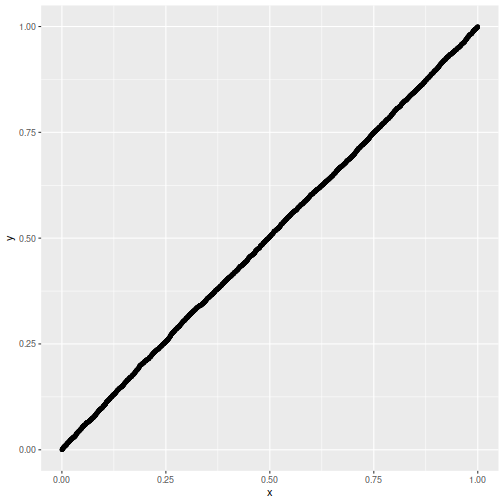Lastly we make one more modification, filling in sample estimates for $$\zeta$$ and $$R$$ into the computation of the covariance. We compute one-sided 95% confidence intervals, and check how the empirical rate of violations. We find the rate to be around 5%. nsim <- 5000 p <- 20 ndays <- 3 * 252 A1 <- cbind(-1,diag(p-1)) set.seed(9873) # 5678 gives exactly 250 / 5000, which is eerie tgtval <- 0.95 viols <- replicate(nsim,{ # population values here mu <- rnorm(p) Sigma <- rWish(n=2*p+5,p=p) RRR <- cov2cor(Sigma) zeta <- mu /sqrt(diag(Sigma)) Xrets <- rmvnorm(ndays,mean=mu,sigma=Sigma) srs <- colMeans(Xrets) / apply(Xrets,2,FUN=sd) Sighat <- cov(Xrets) Rhat <- cov2cor(Sighat) y <- srs # now use the sample approximations. # you can compute this from the observed information. mySigma <- (1/ndays) * (Rhat + (1/2) * diag(srs) %*% (Rhat * Rhat) %*% diag(srs)) # collect the maximum, so reorder the A above yord <- order(y,decreasing=TRUE) revo <- seq_len(p) revo[yord] <- revo A <- A1[,revo] nu <- rep(0,p) nu[yord] <- 1 b <- rep(0,p-1) # mu is unknown to this guy foo <- citn(p=tgtval,y=y,A=A,b=b,nu=nu,Sigma=mySigma) violated <- zeta[yord] < foo }) print(sprintf('%.2f%%',100*mean(viols)))  ##  "5.14%"  ## Putting it together Lee's method appears to give nominal coverage for hypothesis tests and confidence intervals on the SNR of the asset with maximal Sharpe. In principle it should not be affected by correlation of the assets or by large differences in the SNRs of the assets. It should be applicable in the $$p > n$$ case, as we are not inverting the covariance matrix. On the negative side, requiring one estimate the correlation of assets for the computation will not scale with large $$p$$. We are guardedly optimistic that this method is not adversely affected by the normal approximation of the Sharpe ratio, although it would be ill-advised to use it for the case of small samples until more study is performed. Moreover the quantile function we hacked together here should be improved for stability and accuracy. Click to read and post comments #### Jul 14, 2018 ## Distribution of Maximal Sharpe, the Markowitz Approximation In a previous blog post we looked at a statistical test for overfitting of trading strategies proposed by Lopez de Prado, which essentially uses a $$t$$-test threshold on the maximal Sharpe of backtested returns based on assumed independence of the returns. (Actually it is not clear if Lopez de Prado suggests a $$t$$-test or relies on approximate normality of the $$t$$, but they are close enough.) In that blog post, we found that in the presence of mutual positive correlation of the strategies, the test would be somewhat conservative. It is hard to say just how conservative the test would be without making some assumptions about the situations in which it would be used. This is a trivial point, but needs to be mentioned: to create a useful test of strategy overfitting, one should consider how strategies are developed and overfit. There are numerous ways that trading strategies are, or could be developed. I will enumerate some here, roughly in order of decreasing methodological purity: 1. Alice the Quant goes into the desert on a Vision Quest. She emerges three days later with a fully formed trading idea, and backtests it a single time to satisfy the investment committee. The strategy is traded unconditional on the results of that backtest. 2. Bob the Quant develops a black box that generates, on demand, a quantitative trading strategy, and performs a backtest on that strategy to produce an unbiased estimate of the historical performance of the strategy. All strategies are produced de novo, without any relation to any other strategy ever developed, and all have independent returns. The black box can be queried ad infinitum. (This is essentially Lopez de Prado's assumed mode of development.) 3. The same as above, but the strategies possibly have correlated returns, or were possibly seeded by published anomalies or trading ideas. 4. Carole the Quant produces a single new trading idea, in a white box, that is parametrized by a number of free parameters. The strategy is backtested on many settings of those parameters, which are chosen by some kind of design, and the settings which produce the maximal Sharpe are selected. 5. The same as above, except the parameters are optimized based on backtested Sharpe using some kind of hill-climbing heuristic or an optimizer. 6. The same as above, except the trading strategy was generally known and possibly overfit by other parties prior to publication as "an anomaly". 7. Doug the Quant develops a gray box trading idea, adding and removing parameters while backtesting the strategy and debugging the code, mixing machine and human heuristics, and leaving no record of the entire process. 8. A small group of Quants separately develop a bunch of trading strategies, using common data and tools, but otherwise independently hillclimb the in-sample Sharpe, adding and removing parameters, each backtesting countless unknown numbers of times, all in competition to have money allocated to their strategies. 9. The same, except the fund needs to have a 'good quarter', otherwise investors will pull their money, and they really mean it this time. The first development mode is intentionally ludicrous. (In fact, these modes are also roughly ordered by increasing realism.) It is the only development model that might result in underfitting. The division between the second and third modes is loosely quantifiable by the mutual correlation among strategies, as considered in the previous blog post. But it is not at all clear how to approach the remaining development modes with the maximal Sharpe statistic. Perhaps a "number of pseudo-independent backtests" could be estimated and then used with the proposed test, but one cannot say how this would work with in-sample optimization, or the diversification benefit of looking in multidimensional parameter space. ## The Markowitz Approximation Perhaps the maximal Sharpe test can be salvaged, but I come to bury Caesar, not to resuscitate him. Some years ago, I developed a test for overfitting based on an approximate portfolio problem. I am ashamed to say, however, that while writing this blog post I have discovered that this approximation is not as accurate as I had remembered! It is interesting enough to present, I think, warts and all. Suppose you could observe the time series of backtested returns from all the backtests considered. By 'all', I want to be very inclusive if the parameters were somehow optimized by some closed form equation, say. Let $$Y$$ be the $$n \times k$$ matrix of returns, with each row a date, and each column one of the backtests. We suppose we have selected the strategy which maximizes Sharpe, which corresponds to picking the column of $$Y$$ with the largest Sharpe. Now perform some kind of dimensionality reduction on the matrix $$Y$$ to arrive at $$Y \approx X W,$$ where $$X$$ is an $$n \times l$$ matrix, and $$W$$ is an $$l \times k$$ matrix, and where $$l \ll k$$. The columns of $$X$$ approximately span the columns of $$Y$$. Picking the strategy with maximal Sharpe now approximately corresponds to picking a column of $$W$$ that has the highest Sharpe when multiplied by $$X$$. That is, our original overfitting approximately corresponded to the optimization problem $$\max_{w \in W} \operatorname{Sharpe}\left(X w\right).$$ The unconstrained version of this optimization problem is solved by the Markowitz portfolio. Moreover, if the returns $$X$$ are multivariate normal with independent rows, then the distribution of the (squared) Sharpe of the Markowitz portfolio is known, both under the null hypothesis (columns of $$X$$ are all zero mean), and the alternative (the maximal achievable population Sharpe is non-zero), via Hotelling's $$T^2$$ statistic. If $$\hat{\zeta}$$ is the (in-sample) Sharpe of the (in-sample) Markowitz portfolio on $$X$$, assumed i.i.d. Normal, then $$\frac{(n-l) \hat{\zeta}^2}{l (n - 1)}$$ follows an F distribution with $$l$$ and $$n-l$$ degrees of freedom. I wrote the psropt and qsropt functions in SharpeR to compute the CDF and quantile of the maximal in-sample Sharpe to support this kind of analysis. I should note there are a few problems with this approximation: 1. There is no strong theoretical basis for this approximation: we do not have a model for how correlated returns should arise for a particular population, nor what the dimension $$l$$ should be, nor what to expect under the alternative, when the true optimal strategy has positive Sharpe. (I suspect that posing overfit of backtests as a Gaussian Process might be fruitful.) 2. We have to estimate the dimensionality, $$l$$, which is about as odious as estimating the number of 'pseudo-observations' in the maximal Sharpe test. I had originally suspected that $$l$$ would be 'obvious' from the application, but this is not apparently so. 3. Although the returns may live nearly in an $$l$$ dimensional subspace, we might have have selected a suboptimal combination of them in our overfitting process. This would be of no consequence if the $$l$$ were accurately estimated, but it will stymie our testing of the approximation. Despite these problems, let us press on. ### An example: a two window Moving Average Crossover While writing this blog post, I went looking for examples of 'classical' technical strategies which would be ripe for overfitting (and which I could easily simulate under the null hypothesis). I was surprised to find that freely available material on Technical Analysis was even worse than I could imagine. owhere among the annotated plots with silly drawings could I find a concrete description of a trading strategy, possibly with free parameters to be fit to the data. Rather than wade through that swamp any longer, I went with an old classic, the Moving Average Crossover. The idea is simple: compute two moving averages of the price series with different windows. When one is greater than the other, hold the asset long, otherwise hold it short. The choice of two windows must be overfit by the quant. Here I perform that experiment, but under the null hypothesis, with zero mean simulated returns generated independently of each other. Any realization of this strategy, with any choice of the windows, will have zero mean returns and thus zero Sharpe. First I collect 'backtests' (sans any trading costs) of two window MAC for a single realization of returns where the two windows were allowed to vary from 2 to around 1000. The backtest period is 5 years of daily data. I compute the singular value decomposition of the returns, then present a scree plot of the singular values. suppressMessages({ library(dplyr) library(fromo) library(svdvis) }) # return time series of *all* backtests backtests <- function(windows,rel_rets) { nwin <- length(windows) nc <- choose(nwin,2) fwd_rets <- dplyr::lead(rel_rets,1) # log returns log_rets <- log(1 + rel_rets) # price series psers <- exp(cumsum(log_rets)) avgs <- lapply(windows,fromo::running_mean,v=psers) X <- matrix(0,nrow=length(rel_rets),ncol=2*nc) idx <- 1 for (iii in 1:(nwin-1)) { for (jjj in (iii+1):nwin) { position <- sign(avgs[[iii]] - avgs[[jjj]]) myrets <- position * fwd_rets X[,idx] <- myrets X[,idx+1] <- -myrets idx <- idx + 1 } } # trim the last row, which has the last NA X <- X[-nrow(X),] X } geomseq <- function(from=1,to=1,by=(to/from)^(1/(length.out-1)),length.out=NULL) { if (missing(length.out)) { lseq <- seq(log(from),log(to),by=log(by)) } else { lseq <- seq(log(from),log(to),by=log(by),length.out=length.out) } exp(lseq) } # which windows to test windows <- unique(ceiling(geomseq(2,1000,by=1.15))) nobs <- ceiling(3 * 252) maxwin <- max(windows) rel_rets <- rnorm(maxwin + 10 + nobs,mean=0,sd=0.01) XX <- backtests(windows,rel_rets) # grab the last nobs rows XX <- XX[(nrow(XX)-nobs+1):(nrow(XX)),] # perform svd blah <- svd(x=XX,nu=11,nv=11) # look at it ph <- svdvis::svd.scree(blah) + labs(x='Singular Vectors',y='Percent Variance Explained') print(ph)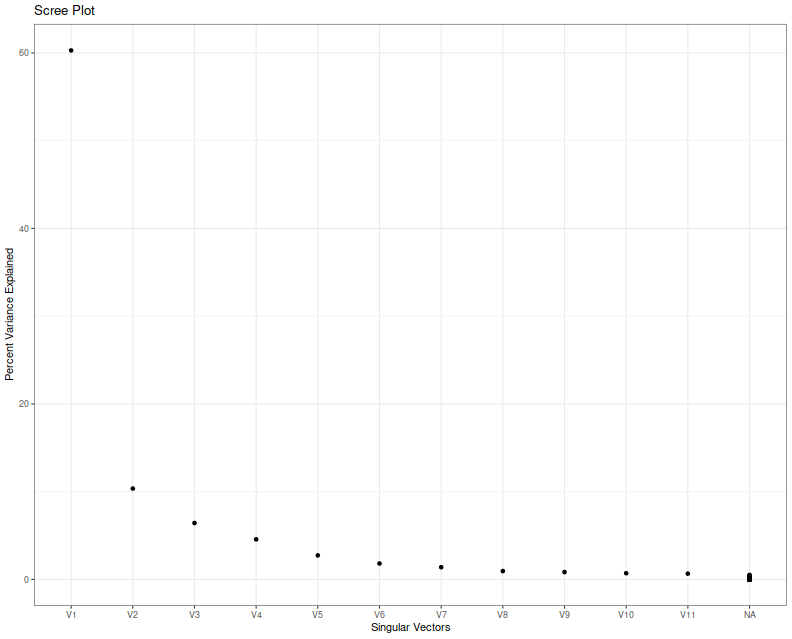I think we can agree that nobody knows how to interpret a scree plot. However, in this case a large proportion of the explained variance seems to encoded in the first two eigenvalues, which is consistent with my a priori guess that $$l=2$$ in this case because of the two free parameters. Next I simulate overfitting, performing that same experiment, but picking the largest in-sample Sharpe ratio. I create a series of independent zero mean returns, then backtest a bunch of MAC strategies, and save the maximal Sharpe over a 3 year window of daily data. I repeat this experiment ten thousand times, and then look at the distribution of that maximal Sharpe. suppressMessages({ library(dplyr) library(tidyr) library(tibble) library(SharpeR) library(future.apply) library(ggplot2) }) ope <- 252 geomseq <- function(from=1,to=1,by=(to/from)^(1/(length.out-1)),length.out=NULL) { if (missing(length.out)) { lseq <- seq(log(from),log(to),by=log(by)) } else { lseq <- seq(log(from),log(to),by=log(by),length.out=length.out) } exp(lseq) } # one simulation. returns maximal Sharpe onesim <- function(windows,n=1000) { maxwin <- max(windows) rel_rets <- rnorm(maxwin + 10 + n,mean=0,sd=0.01) fwd_rets <- dplyr::lead(rel_rets,1) # log returns log_rets <- log(1 + rel_rets) # price series psers <- exp(cumsum(log_rets)) avgs <- lapply(windows,fromo::running_mean,v=psers) nwin <- length(windows) maxsr <- 0 for (iii in 1:(nwin-1)) { for (jjj in (iii+1):nwin) { position <- sign(avgs[[iii]] - avgs[[jjj]]) myrets <- position * fwd_rets # compute Sharpe on some part of this compon <- myrets[(length(myrets)-n):(length(myrets)-1)] thissr <- SharpeR::as.sr(compon,ope=ope)$sr
# we are implicitly testing both combinations of long and short here,
# so we take the absolute Sharpe, since we will always overfit to
# the better of the two:
maxsr <- max(maxsr,abs(thissr))
}
}
maxsr
}

windows <- unique(ceiling(geomseq(2,1000,by=1.15)))
nobs <- ceiling(3 * 252)
nrep <- 10000
plan(multiprocess)
set.seed(1234)
system.time({
simvals <- future_replicate(nrep,onesim(windows,n=nobs))
})

   user  system elapsed
0.722   0.189 245.765


Here I plot the empirical quantiles of the maximal (annualized) Sharpe versus theoretical quantiles under the Markowitz approximation, assuming $$l=2$$. I also plot the $$y=x$$ lines, and horizontal and vertical lines at the nominal upper $$0.05$$ cutoff based on the Markowitz approximation.

# plot max value vs quantile
library(ggplot2)
apxdf <- 2.0
ph <- data.frame(simvals=simvals) %>%
ggplot(aes(sample=simvals)) +
geom_vline(xintercept=SharpeR::qsropt(0.95,df1=apxdf,df2=nobs,zeta.s=0,ope=ope),linetype=3) +
geom_hline(yintercept=SharpeR::qsropt(0.95,df1=apxdf,df2=nobs,zeta.s=0,ope=ope),linetype=3) +
stat_qq(distribution=SharpeR::qsropt,dparams=list(df1=apxdf,df2=nobs,zeta.s=0,ope=ope)) +
geom_abline(intercept=0,slope=1,linetype=2) +
labs(title='empirical quantiles of maximal Sharpe versus Markowitz Approximation',
x='theoretical quantile',y='empirical quantile (Sharpe in annual units)')
print(ph)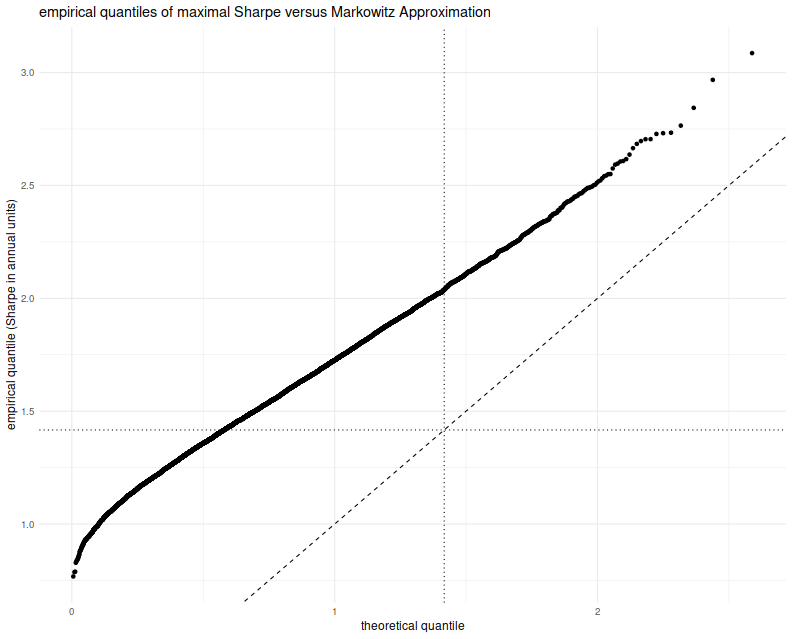This approximation is clearly no good. The empirical rate of type I errors at the $$0.05$$ level is around 60%, and the Q-Q line is just off. I must admit that when I previously looked at this approximation (and in the vignette for SharpeR!) I used the qqline function in base R, which fits a line based on the first and third quartile of the empirical fit. That corresponds to an affine shift of the line we see here, and nothing seems amiss.

So perhaps the Markowitz approximation can be salvaged, if I can figure out why this shift occurs. Perhaps we have only traded picking a maximal $$t$$ for picking a maximal $$T^2$$ and there still has to be a mechanism to account for that. Or perhaps in this case, despite the 'obvious' setting of $$l=2$$, we should have chosen $$l=7$$, for which the empirical rate of type I errors is around 60%, though we have no way of seeing that 7 from the scree plot or by looking at the mechanism for generating strategies. Or perhaps the problem is that we have not actually picked a maximal strategy over the subspace, and this technique can only be used to provide a possibly conservative test. In this regard, our test would be no more useful than the maximal Sharpe test described in the previous blog post.

## Distribution of Maximal Sharpe

Before throwing money at the best strategy, first you have to identify it (probably via the Sharpe ratio on the backtested returns, or some other heuristic objective), then you should probably assess whether it is any good, or simply the result of "dumb luck". More formally, you might perform a hypothesis test under the null hypothesis that all the generated strategies have non-positive expected returns, or you might try to construct a confidence interval on the Signal-Noise ratio of the strategy with best in-sample Sharpe.

The "Most Important Plot" in all finance is, apparently, a representation of the distribution of the maximal in-sample Sharpe ratio of $$B$$ different backtests over strategies that are zero mean, and have independent returns, versus that $$B$$. As presented by its author it is a heatmap, though I imagine boxplots or violin plots would be easier to digest. To generate this plot, note that the smallest value of $$B$$ independent uniform random variates takes a Beta distribution with parameters $$1$$ and $$B$$. So to find the $$q$$th quantile of the maximum Sharpe, compute the $$1-q$$ quantile of the $$\beta\left(1,B\right)$$ distribution, then plug that the quantile function of the Sharpe distribution with the right degrees of freedom and zero Signal Noise parameter. (See Exercise 3.29 in my Short Sharpe Course.)

In theory this should give you a significance threshold against which you can compare your observed maximal Sharpe. If yours is higher, presumably you should trade the strategy (uhh, after you pay Marcos for using his patented technology), otherwise give up quantitative trading and become an accountant. One huge problem with this Most Important Plot method (besides its ignorance of entire fields of research on Multiple Hypothesis Testing, False Discovery Rate, Estimation After Selection, White's Reality Check, Romano-Wolf, Politis, Hansen's SPA, inter alia) is the assumption of independence. "Assuming quantities are independent which are not independent" is the downfall of many a statistical procedure applied in the wild (much more so than non-normality), and here is no different. And we have plenty of reason to believe returns from any real strategy generation process would fail independence:

1. Strategies are typically generated on a limited universe of assets, and using a limited set of predictive 'features', and are tested on a single, often relatively short, history.
2. Most strategy generation processes (synthetic and human) have a very limited imagination.
3. Most strategy generation processes (synthetic and human) tend to work incrementally, generating new strategies after having observed the in-sample performance of existing strategies. They "hill-climb".

My initial intuition was that dependence among strategies, especially of the hill-climbing variety, would cause this Most Important Test to have a much higher rate of type I errors than advertised. (This would be bad, since it would effectively still pass dud trading strategies while selling you a false sense of security.) However, it seems that the more innocuous correlation of random generation on a limited set of strategies and assets causes this test to be conservative. (It is similar to Bonferroni's correction in this regard.)

To establish this conservatism, you can use Slepian's Lemma. This lemma is a kind of stochastic dominance result for multivariate normals. It says that if $$X$$ and $$Y$$ are $$B$$-variate normal random variables, where each element is zero mean and unit variance, and if the covariance of any pair of elements of $$X$$ is no less than the covariance of the corresponding pair of elements of $$Y$$, then $$Y$$ stochastically dominates $$X$$, in the multivariate sense. This is actually a stronger result than what we need, which is stochastic dominance of the maximal element of $$Y$$ over the maximal element of $$X$$, which it implies.

Here I simply illustrate this dominance empirically. I create a $$B$$-variate normal with zero mean and unit variance of marginals, for $$B=1000$$. The elements are all correlated to each other with correlation $$\rho$$. I compute the maximum over the $$B$$ elements. I perform this simulation 100 thousand times, then compute the $$q$$th empirical quantile over the $$10^5$$ maximum values. I vary $$\rho$$ from 0 to 0.75. Here is the code:

suppressMessages({
library(dplyr)
library(tidyr)
library(tibble)
library(doFuture)
library(broom)
library(ggplot2)
})
# one (bunch of) simulation.
onesim <- function(B,rho=0,propcor=1.0,nsims=100L) {
propcor <- min(1.0,max(propcor,1-propcor))
rho <- abs(rho)
# (anti)correlated part; each row is a simulation
X0 <- outer(array(rnorm(nsims)),array(2*rbinom(B,size=1,prob=propcor)-1))
# idiosyncratic part
XF <- matrix(rnorm(B*nsims),nrow=nsims,ncol=B)
XX <- sqrt(rho) * X0 + sqrt(1-rho) * XF
data_frame(maxval=apply(XX,1,FUN="max"))
}
# many sims.
repsim <- function(B,rho=0,propcor=1.0,nsims=1000L) {
maxper <- 100L
nreps <- ceiling(nsims / maxper)
jumble <- replicate(nreps,onesim(B=B,rho=rho,propcor=propcor,nsims=maxper),simplify=FALSE) %>%
bind_rows()
}
manysim <- function(B,rho=0,propcor=1.0,nsims=10000L,nnodes=7) {
if ((nsims > 100*nnodes) && require(doFuture)) {
registerDoFuture()
plan(multiprocess)
# do in parallel.
nper <- ceiling(nsims / nnodes)
retv <- foreach(i=1:nnodes,.export = c('B','rho','propcor','nper','onesim','repsim')) %dopar% {
repsim(B=B,rho=rho,propcor=propcor,nsims=nper)
} %>%
bind_rows()
retv <- retv[1:nsims,]
} else {
retv <- repsim(B=B,rho=rho,propcor=propcor,nsims=nsims)
}
retv
}

params <- tidyr::crossing(data_frame(rho=c(0,0.25,0.5,0.75)),
data_frame(propcor=c(0.5,1.0))) %>%
filter(rho > 0 | propcor == 1)

# run a bunch;
nrep <- 1e5
set.seed(1234)
system.time({
results <- params %>%
group_by(rho,propcor) %>%
summarize(sims=list(manysim(B=1000,rho=rho,propcor=propcor,nsims=nrep))) %>%
ungroup() %>%
tidyr::unnest()
})

   user  system elapsed
142.680   3.522  27.266

# aggregate the results
do_aggregate <- function(results) {
results %>%
group_by(rho,propcor) %>%
summarize(qs=list(broom::tidy(quantile(maxval,probs=seq(0.50,0.9975,by=0.0025))))) %>%
ungroup() %>%
tidyr::unnest() %>%
rename(qtile=names,value=x)
}
sumres <- results %>% do_aggregate()


Here I plot the empirical $$q$$th quantile of the maximum versus $$q$$, with different lines for the different values of $$\rho$$. I include a vertical line at the 0.95 quantile, to show where the nominal 0.05 level threshold is. The takeaway, as implied by Slepian's lemma, is that the maximum over $$B$$ Gaussian elements decreases stochastically as the correlation of elements increases. Thus when you assume independence of your backtests, you use the red line as your significance threshold (typically where it intersects the dashed vertical line), while your processes live on the green or blue or purple lines. Your test will be too conservative, and your true type I rate will be lower than the nominal rate.

# plot max value vs quantile
library(ggplot2)
ph <- sumres %>%
filter(propcor==1) %>%
mutate(rho=factor(rho)) %>%
mutate(xv=as.numeric(gsub('%$','',qtile))) %>% ggplot(aes(x=xv,y=value,color=rho,group=rho)) + geom_line() + geom_point(alpha=0.2) + geom_vline(xintercept=95,linetype=2,alpha=0.5) + # the 0.05 significance cutoff labs(title='quantiles of maximum of B=1000 multivariate normal, various correlations', x='quantile',y='maximum value') print(ph)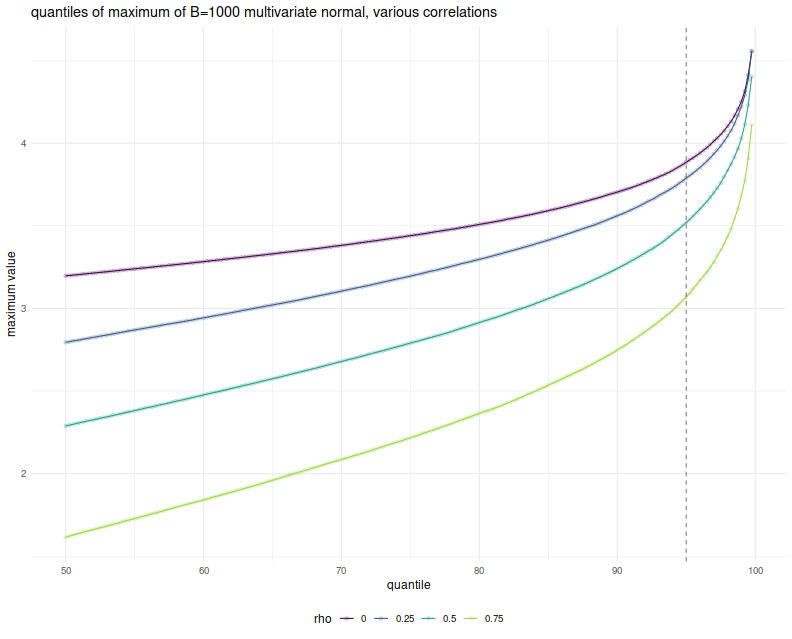But wait, these simulations were over Gaussian vectors (and Slepian's Lemma is only applicable in the Gaussian case), while the Most Important Test is to be applied to the Sharpe ratio. They have different distributions. It turns out, however, that when the population mean is the zero vector, the vector of Sharpe ratios of returns with correlation matrix $$R$$ is approximately normal with variance-covariance matrix $$\frac{1}{n} R$$. (This is in section 4.2 of Short Sharpe Course.) Here I establish that empirically, by performing the same simulations again, this time spawning 252 days of normally distributed returns with zero mean, for $$B$$ possibly correlated strategies, computing their Sharpes, and taking the maximum. I only perform $$10^4$$ simulations because this one is quite a bit slower: # really just one simulation. onesim <- function(B,nday,rho=0,propcor=1.0,pzeta=0) { propcor <- min(1.0,max(propcor,1-propcor)) rho <- abs(rho) # correlated part; each row is one day X0 <- outer(array(rnorm(nday)),array(2*rbinom(B,size=1,prob=propcor)-1)) # idiosyncratic part XF <- matrix(rnorm(B*nday),nrow=nday,ncol=B) XX <- pzeta + (sqrt(rho) * X0 + sqrt(1-rho) * XF) sr <- colMeans(XX) / apply(XX,2,FUN="sd") # if you wanted to look at them cumulatively: #data_frame(maxval=cummax(sr),iterate=1:B) # otherwise just the maxval data_frame(maxval=max(sr),iterate=B) } # many sims. repsim <- function(B,nday,rho=0,propcor=1.0,pzeta=0,nsims=1000L) { jumble <- replicate(nsims,onesim(B=B,nday=nday,rho=rho,propcor=propcor,pzeta=pzeta),simplify=FALSE) %>% bind_rows() } manysim <- function(B,nday,rho=0,propcor=1.0,pzeta=0,nsims=1000L,nnodes=7) { if ((nsims > 10*nnodes) && require(doFuture)) { registerDoFuture() plan(multiprocess) # do in parallel. nper <- as.numeric(table(1:nsims %% nnodes)) retv <- foreach(iii=1:nnodes,.export = c('B','nday','rho','propcor','pzeta','nper','onesim','repsim')) %dopar% { repsim(B=B,nday=nday,rho=rho,propcor=propcor,pzeta=pzeta,nsims=nper[iii]) } %>% bind_rows() } else { retv <- repsim(B=B,nday=nday,rho=rho,propcor=propcor,pzeta=pzeta,nsims=nsims) } retv } params <- tidyr::crossing(data_frame(rho=c(0,0.25,0.5,0.75)), data_frame(propcor=c(1.0))) %>% filter(rho > 0 | propcor == 1) # run a bunch; nday <- 252 numbt <- 1000 nrep <- 1e4 # should take around 8 minutes on 7 cores set.seed(1234) system.time({ sh_results <- params %>% group_by(rho,propcor) %>% summarize(sims=list(manysim(B=numbt,nday=nday,propcor=propcor,pzeta=0,rho=rho,nsims=nrep))) %>% ungroup() %>% tidyr::unnest() })   user system elapsed 2314.764 3.207 459.758  # aggregate sh_sumres <- sh_results %>% filter(iterate==1000) %>% do_aggregate() %>% mutate(value=sqrt(252) * value) # annualize!  Again, I plot the empirical $$q$$th quantile of the maximum Sharpe, in annualized units, versus $$q$$, with different lines for the different values of $$\rho$$. Because we take one year of returns and annualize the Sharpe, the test statistics should be approximately normal with approximately unit marginal variances. This plot should look eerily similar to the one above, so I overlay the Sharpe simulation results with the results of the Gaussian experiment above to show how close they are. Very little has been lost in the normal approximation to the sample Sharpe, but the maximal Sharpes are slightly elevated compared to the Gaussian case. # plot max value vs quantile library(ggplot2) ph <- sh_sumres %>% mutate(simulation='sharpe') %>% rbind(sumres %>% mutate(simulation='gaussian')) %>% filter(propcor==1) %>% mutate(rho=factor(rho)) %>% mutate(xv=as.numeric(gsub('%$','',qtile))) %>%
ggplot(aes(x=xv,y=value,color=rho,linetype=simulation)) +
geom_line() + geom_point(alpha=0.2) +
geom_vline(xintercept=95,linetype=2,alpha=0.5) +    # the 0.05 significance cutoff
labs(title='maximum versus quantile',x='quantile',y='maximum value')
print(ph)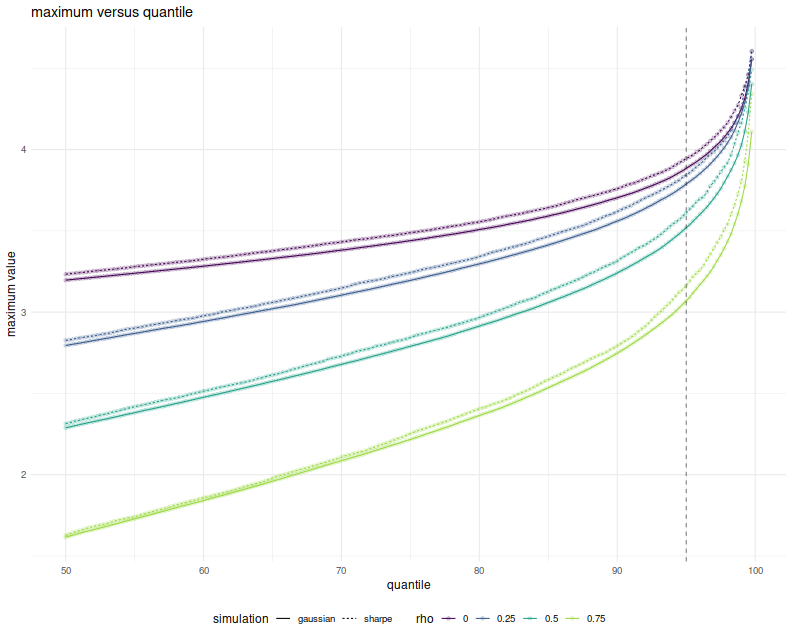The simulations here show what can happen when many strategies have mutual positive correlation. One might wonder what would happen if there were many strategies with a significant negative correlation. It turns out this is not really possible. In order for the correlation matrix to be positive definite, you cannot have too many strongly negative off-diagonal elements. Perhaps there is a Pigeonhole Principle argument for this, but a simple expectation argument suffices.

Just in case, however, above I also simulated the case where you flip a coin to determine whether an element of the Gaussian has a positive or negative correlation to the common element. When the coin is completely biased to heads, you get the simulations shown above. When the coin is fair, the elements of the Gaussian are expected to be divided evenly into two highly groups. Here is the plot of the $$q$$th quantile of the maximum versus $$q$$, with different colors for $$\rho$$ and different lines for the probability of heads. Allowing negatively correlated elements does stochastically increase the maximum element, but never above the $$\rho=0$$ case. And so the Most Important Test still appears conservative.

# plot max value vs quantile
library(ggplot2)
ph <- sumres %>%
mutate(rho=factor(rho)) %>%
mutate(prob. heads=factor(propcor)) %>%
mutate(xv=as.numeric(gsub('%\$','',qtile))) %>%
ggplot(aes(x=xv,y=value,color=rho,linetype=prob. heads)) +
geom_line() + geom_point(alpha=0.2) +
geom_vline(xintercept=95,linetype=2,alpha=0.5) +    # the 0.05 significance cutoff
labs(title='quantiles of maximum of B=1000 multivariate normal, various (anti) correlations',
x='quantile',y='maximum value')
print(ph)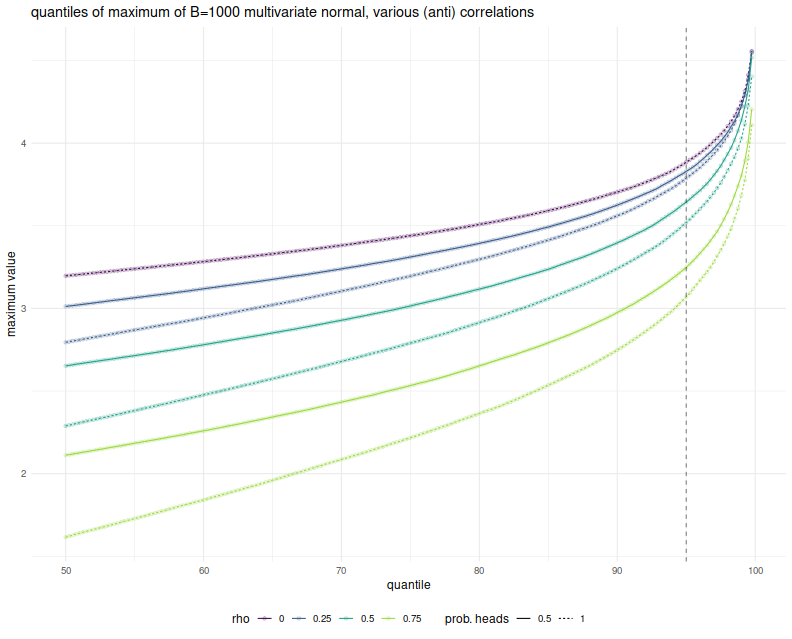In a followup post I will attempt to address the conservatism and hill-climbing issues, using the Markowitz approximation.